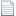# 提升方法

1. 多轮训练，多个分类器

2. 每轮训练增加错误分类样本的权值，降低正确分类样本的权值

3. 降低错误率高的分类器的权值，增加正确率高的分类器的权值(1)初始化训练数据的权值分布(2)（这里的M原著未做解释，其实是表示训练的迭代次数，是由用户指定的。每轮迭代产生一个分类器，最终就有M个分类器）：

(a)使用具有权值分布的训练数据集学习，得到基本分类器(b)计算在训练数据集上的分类误差率(c）计算的系数(d)更新训练数据集的权值分布y只有正负一两种取值，所以上式可以写作：(3)构建基本分类器的线性组合### 前向分步算法(1)初始化(2)（a极小化损失函数(b)更新(3)得到加法模型## 提升树### 提升树算法## 提升树的Python实现

### 测试数据

```def loadSimpData():
"""

:return:
"""
datMat = matrix([[1., 2.1],
[2., 1.1],
[1.3, 1.],
[1., 1.],
[2., 1.]])
classLabels = [1.0, 1.0, -1.0, -1.0, 1.0]
return datMat, classLabels```

```def plotData(datMat, classLabels):
xcord0 = []
ycord0 = []
xcord1 = []
ycord1 = []

for i in range(len(classLabels)):
if classLabels[i]==1.0:
xcord1.append(datMat[i,0]), ycord1.append(datMat[i,1])
else:
xcord0.append(datMat[i,0]), ycord0.append(datMat[i,1])
fig = plt.figure()
ax.scatter(xcord0,ycord0, marker='s', s=90)
ax.scatter(xcord1,ycord1, marker='o', s=50, c='red')
plt.title('decision stump test data')
plt.show()```### 单层决策树分类

```def stumpClassify(dataMatrix, dimen, threshVal, threshIneq):  # just classify the data
"""

:param dataMatrix: 数据
:param dimen: 特征的下标
:param threshVal: 阈值
:param threshIneq: 大于或小于
:return: 分类结果
"""
retArray = ones((shape(dataMatrix), 1))
if threshIneq == 'lt':
retArray[dataMatrix[:, dimen] <= threshVal] = -1.0
else:
retArray[dataMatrix[:, dimen] > threshVal] = -1.0
return retArray```

dimen决定了这个树桩能用的唯一特征。

### 构建决策树桩

```def buildStump(dataArr, classLabels, D):
"""

:param dataArr: 数据特征矩阵
:param classLabels: 标签向量
:param D: 训练数据的权重向量
:return: 最佳决策树,最小的错误率加权和,最优预测结果
"""
dataMatrix = mat(dataArr)
labelMat = mat(classLabels).T
m, n = shape(dataMatrix)
numSteps = 10.0
bestStump = {}
bestClasEst = mat(zeros((m, 1)))
minError = inf  # 将错误率之和设为正无穷
for i in range(n):  # 遍历所有维度
rangeMin = dataMatrix[:, i].min()   #该维的最小最大值
rangeMax = dataMatrix[:, i].max()
stepSize = (rangeMax - rangeMin) / numSteps
for j in range(-1, int(numSteps) + 1):  # 遍历这个区间
for inequal in ['lt', 'gt']:  # 遍历大于和小于
threshVal = (rangeMin + float(j) * stepSize)
predictedVals = stumpClassify(dataMatrix, i, threshVal,
inequal)  # 使用参数 i, j, lessThan 调用树桩决策树分类
errArr = mat(ones((m, 1)))
errArr[predictedVals == labelMat] = 0 # 预测正确的样本对应的错误率为0,否则为1
weightedError = D.T * errArr  # 计算错误率加权和
# print "split: dim %d, thresh %.2f, thresh ineqal: %s, the weighted error is %.3f" % (i, threshVal, inequal, weightedError)
if weightedError < minError:    # 记录最优树桩决策树分类器
minError = weightedError
bestClasEst = predictedVals.copy()
bestStump['dim'] = i
bestStump['thresh'] = threshVal
bestStump['ineq'] = inequal
return bestStump, minError, bestClasEst```

`weightedError = D.T * errArr  # 计算错误率加权和````def adaBoostTrainDS(dataArr, classLabels, numIt=40):
"""

:param dataArr: 样本特征矩阵
:param classLabels: 样本分类向量
:param numIt: 迭代次数
:return: 一系列弱分类器及其权重,样本分类结果
"""
weakClassArr = []
m = shape(dataArr)
D = mat(ones((m, 1)) / m)  # 将每个样本的权重初始化为均等
aggClassEst = mat(zeros((m, 1)))
for i in range(numIt):
bestStump, error, classEst = buildStump(dataArr, classLabels, D)  # 构建树桩决策树,这是一个若分类器,只能利用一个维度做决策
# print "D:",D.T
alpha = float(
0.5 * log((1.0 - error) / max(error, 1e-16)))  # 计算 alpha, 防止发生除零错误
bestStump['alpha'] = alpha
weakClassArr.append(bestStump)  # 保存树桩决策树
# print "classEst: ",classEst.T
expon = multiply(-1 * alpha * mat(classLabels).T, classEst)  # 每个样本对应的指数,当预测值等于y的时候,恰好为-alpha,否则为alpha
D = multiply(D, exp(expon))  # 计算下一个迭代的D向量
D = D / D.sum() # 归一化
# 计算所有分类器的误差,如果为0则终止训练
aggClassEst += alpha * classEst
# print "aggClassEst: ",aggClassEst.T
aggErrors = multiply(sign(aggClassEst) != mat(classLabels).T, ones((m, 1))) # aggClassEst每个元素的符号代表分类结果,如果与y不等则表示错误
errorRate = aggErrors.sum() / m
print "total error: ", errorRate
if errorRate == 0.0: break
return weakClassArr, aggClassEst```

```        alpha = float(
0.5 * log((1.0 - error) / max(error, 1e-16)))  # 计算 alpha, 防止发生除零错误``````        expon = multiply(-1 * alpha * mat(classLabels).T, classEst)  # 每个样本对应的指数,当预测值等于y的时候,恰好为-alpha,否则为alpha
D = multiply(D, exp(expon))  # 计算下一个迭代的D向量
D = D / D.sum() # 归一化```### 提升树分类

```def adaClassify(datToClass, classifierArr):
dataMatrix = mat(datToClass)
m = shape(dataMatrix)
aggClassEst = mat(zeros((m, 1)))
for i in range(len(classifierArr)):
classEst = stumpClassify(dataMatrix, classifierArr[i]['dim'], \
classifierArr[i]['thresh'], \
classifierArr[i]['ineq'])
aggClassEst += classifierArr[i]['alpha'] * classEst
print aggClassEst
return sign(aggClassEst)```

### 调用方法

```datArr, labelArr = loadSimpData()
classifierArr, aggClassEst = adaBoostTrainDS(datArr, labelArr, 30)

```total error:  0.2
total error:  0.2
total error:  0.0
[[-0.69314718]]
[[-1.66610226]]
[[-2.56198199]]
[[-1.]]```

```total error:  0.2
total error:  0.2
total error:  0.0```### 复杂数据集

《机器学习实战》还给出了一个马疝病数据集：horseColicTraining2.txt

```def loadDataSet(fileName):
"""
从文件中加载以制表符分割的数据集(浮点数格式)
:param fileName:
:return:
"""
dataMat = []
labelMat = []
fr = open(fileName)
lineArr = []
curLine = line.strip().split('\t')
for i in range(numFeat - 1):
lineArr.append(float(curLine[i]))
dataMat.append(lineArr)
labelMat.append(float(curLine[-1]))
return dataMat, labelMat```

```datArr, labelArr = loadDataSet("horseColicTraining2.txt")
classifierArr, aggClassEst = adaBoostTrainDS(datArr, labelArr, 10)```

### 准确率与召回率`TP / (TP + FP)`

`TP / (TP + FN)`

```def evaluate(aggClassEst, classLabels):
"""

:param aggClassEst:
:param classLabels:
:return: P, R
"""
TP = 0.
FP = 0.
TN = 0.
FN = 0.
for i in range(len(classLabels)):
if classLabels[i] == 1.0:
if (sign(aggClassEst[i]) == classLabels[i]):
TP += 1.0
else:
FP += 1.0
else:
if (sign(aggClassEst[i]) == classLabels[i]):
TN += 1.0
else:
FN += 1.0

return TP / (TP + FP), TP / (TP + FN)

def train_test(datArr, labelArr, datArrTest, labelArrTest, num):
classifierArr, aggClassEst = adaBoostTrainDS(datArr, labelArr, num)
prTrain = evaluate(aggClassEst, labelArr)
prTest = evaluate(aggClassEst, labelArrTest)
return prTrain, prTest

prTrain, prTest = train_test(datArr, labelArr, datArrTest, labelArrTest, 10)
print prTrain, prTest```

`(0.8595505617977528, 0.7766497461928934) (0.7872340425531915, 0.8604651162790697)`### ROC曲线

```def plotROC(predStrengths, classLabels):
import matplotlib.pyplot as plt
cur = (1.0, 1.0)  # 中间变量,初始状态为右上角
ySum = 0.0  # variable to calculate AUC
numPosClas = sum(array(classLabels) == 1.0)  # TP
yStep = 1 / float(numPosClas)
xStep = 1 / float(len(classLabels) - numPosClas)
sortedIndicies = predStrengths.argsort()  # 按元素值排序后的下标,逆序
fig = plt.figure()
fig.clf()
ax = plt.subplot(111)
# 遍历所有的值
for index in sortedIndicies.tolist():
if classLabels[index] == 1.0:  # 预测正确
delX = 0  # 真阳率不变
delY = yStep  # 假阳率减小
else:
delX = xStep
delY = 0
ySum += cur  # ROC面积的一个小长条
# 从 cur 到 (cur-delX,cur-delY) 画一条线
ax.plot([cur, cur - delX], [cur, cur - delY], c='b')
cur = (cur - delX, cur - delY)
ax.plot([0, 1], [0, 1], 'b--')  # 随机猜测的ROC线
plt.xlabel('False positive rate')
plt.ylabel('True positive rate')
plt.title('ROC curve for AdaBoost horse colic detection system')
ax.axis([0, 1, 0, 1])
plt.show()
print "the Area Under the Curve is: ", ySum * xStep
plotROC(aggClassEst.T, labelArr)```《统计学习方法》

《机器学习实战》

1. #2